# GSEB Solutions Class 9 Maths Chapter 12 Heron’s Formula Ex 12.2

Gujarat Board GSEB Solutions Class 9 Maths Chapter 12 Heron’s Formula Ex 12.2 Textbook Questions and Answers.

## Gujarat Board Textbook Solutions Class 9 Maths Chapter 12 Heron’s Formula Ex 12.2

Question 1.
A park in the shape of a quadrilateral ABCD, has ∠C = 90°,AB = 9m, BC = 12m,CD = 5m and AD = 8 m. How much area does it occupy?
Solution:
Join BD.
Area of right triangle BCD
= $$\frac {1}{2}$$ x base x height
= $$\frac {1}{2}$$ x 5 x 12 = 30m2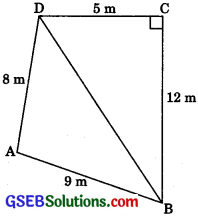In right triangle BCD,
BD2 = BC2 + CD2 (By Pythagoras Theorem)
= (12)2 + (5)2 = 144 + 25 = 169
= BD = $$\sqrt{169}$$ = 13m
For ΔABD
a = 13m, b = 8m, c = 9m
∴ s = $$\frac {a + b + c}{2}$$
s = $$\frac {13 + 8 + 9}{2}$$ = $$\frac {30}{2}$$ = 15m
∴ Area of the ΔABD
= $$\sqrt{s(s-a)(s-b)(s-c)}$$
= $$\sqrt{15(15-13)(15-8)(15-9)}$$
= $$\sqrt{15(2)(7)(6)}$$
= $$\sqrt{(3 \times 5)(2)(7)(2 \times 3)}$$
= 3 x 2$$\sqrt{(35}$$ = 6$$\sqrt{(35}$$m2
= 6 x 5.916 = 35.5 m2 (approx.)

∴ Area of the quadrilateral ΔBCD
= Area of ΔBCD + Area of ΔABD
= 30 m2 + 35.5 m2
= 65.5 m2 (approx.)
Hence, the park occupies the area 65.5 m2 (approx.).Question 2.
Find the area of a quadrilateral ABCD in which AB = 3 cm, BC = 4 cm, CD = 4 cm, DA = 5 cm and AC =5cm.
Solution:
For ΔABC
a = 4cm, b = 5cm, c = 3cm
∴ a2 + c2 = b2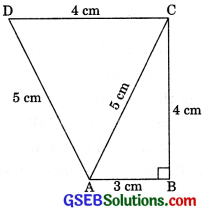ΔABC is right angled with ∠B = 90
∴ Area of right triangle ABC
= $$\frac {1}{2}$$ x base x height
= $$\frac {1}{2}$$ x 3 x 4 = 6 cm2
For ΔACD
a = 4 cm, b = 5 cm, c = 5 cm
s = $$\frac {a + b + c}{2}$$ = $$\frac {4 + 5 + 5}{2}$$ = $$\frac {14}{2}$$ = 7cm
∴ Area of the ACD
= $$\sqrt{s(s-a)(s-b)(s-c)}$$
= $$\sqrt{7(7-4)(7-5)(7-5)}$$
= .$$\sqrt{7(3)(2)(2)}$$ = 2 $$\sqrt{21}$$cm2
= 2 x 4.6 cm2 (approx.)
= 9.2 cm2 (approx.)
∴ Area of the quadrilateral ABCD
= Area of ΔABC + Area of ΔACD
= 6 cm2 + 9.2 cm2
= 15.2 cm2 (approx.)∴ Area of the quadrilateral ABCD
= Area of ÊABC + Area of ΔACD
= 6 cm2 + 9.2 cm2
= 15.2 cm2 (approx.)

Question 3.
Radha made a picture of an aeroplane with colored paper as shown in figure. Find the total area of the paper used.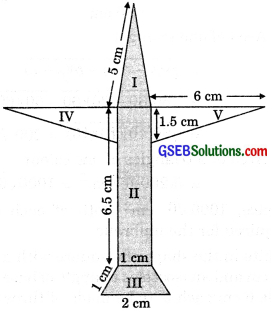Solution:
For triangular Area-I
a = 5 cm, b = 5cm, c = 1cm
s = $$\frac {a + b + c}{2}$$ = $$\frac {5 + 5 + 1}{2}$$ = $$\frac {11}{2}$$ = 5.5 cm
∴ Area-I = $$\sqrt{s(s-a)(s-b)(s-c)}$$
= $$\sqrt{5.5(5.5-5)(5.5-5)(5.5-1)}$$
= $$\sqrt{5.5(.5)(.5)(4.5)}$$
= $$(.5) \sqrt{(5.5)(4.5)}$$
= $$(.5) \sqrt{(.5)(11)(.5)(9)}$$
= (.5) (.5) (3) $$\sqrt{11}$$
= 0.75 $$\sqrt{11}$$ = 0.75(3.3) (approx.)
= 2.5 cm2 (approx.)
Area-II = 6.5 x 1 = 6.5 cm2
For Area-III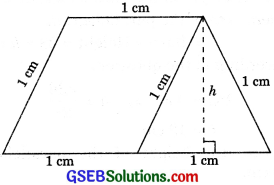Height of an equilateral = $$\frac{\sqrt{3}}{2}$$ a = $$\frac{\sqrt{3}}{2}$$(1)
∴ h = $$\frac{\sqrt{3}}{2}$$ cm
Area of trapezium
= $$\frac {1}{2}$$ x (1 + 2) x $$\frac{\sqrt{3}}{2}$$
= $$\frac{3 \sqrt{3}}{4}$$ = $$\frac {3}{4}$$ x 1.732
= 1.3 cm2 (approx.)

Area-IV= $$\frac{6 \times 1.5}{2}$$ = 4.5 cm2
Area-V= $$\frac{6 \times 1.5}{2}$$ = 4.5 cm2
∴ Total area of the paper used
= Area-I + Area-II + Area-III + Area-IV + Area-V
= 2.5 2cm2 + 6.5 cm2 + 1.3 cm2 + 4.5 cm2 + 4.5 cm2
= 19.3 cm2.Question 4.
A triangle and a parallelogram have the same base and the same area. If the sides of the triangle are 26 cm, 28 cm, and 30 cm, and the parallelogram stands on the base 28 cm, find the height of the parallelogram.
Solution:
For triangle
a = 26 cm, b = 28 cm, c = 30
s = $$\frac{a+b+c}{2}$$
s = $$\frac{26+28+30}{2}$$ = $$\frac{84}{2}$$ = 42 cm

∴ Area of the triangle
= $$\sqrt{s(s-a)(s-b)(s-c)}$$
= $$\sqrt{42(42-26)(42-28)(42-30)}$$
= $$\sqrt{42(16)(14)(12)}$$
= $$\sqrt{(6 \times 7)(16)(7 \times 2)(6 \times 2)}$$
= 6 x 4 x 7 x 2= 336 cm2.
Let the height of the parallelogram be h cm.
Then, area of the parallelogram = Base x Height = 28 x h cm2
According to the question,
28 h = 336 h = $$\frac{336}{28}$$
h = 12 cm
Hence, the height of the parallelogram is 12 cm.Question 5.
A rhombus-shaped field has green grass for 18 cows to graze. If each side of the rhombus is 30 m and its longer diagonal is 48 m, how much area of grass field will each cow be getting?
For ΔABC
a = 30m, b = 48m, c = 30m
s = $$\frac{a+b+c}{2}$$ = $$\frac{30+48+30}{2}$$ = $$\frac{108}{2}$$ = 54 m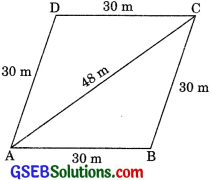∴ Area of ΔABC
= $$\sqrt{s(s-a)(s-b)(s-c)}$$
= $$\sqrt{54(54-30)(54-48)(54-30)}$$
= $$\sqrt{54(24)(6)(24)}$$
= $$\sqrt{(9 \times 6)(24)(6)(24)}$$ = 3 x 6 x 24 = 432 m2

∴ Area of the rhombus
= 2 x area of ΔABC
= 2 x 432 = 864 m2
∴ Area of grass for 18 cows = 864 m2
∴ Area of grass for 1 cow
= $$\frac{864}{18}$$m2 = 48m2Question 6.
An umbrella is made by stitching 10 triangular pieces of cloth of two different colours (see figure), each piece measuring 20 cm, 50 cm and 50 cm. How much cloth of each colour is required for the umbrella?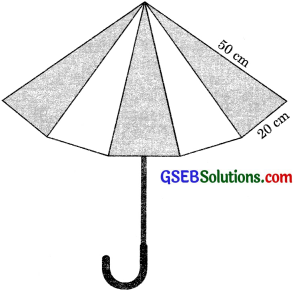Solution:
For one triangular piece
a = 20 cm,b = 50cm, c = 50 cm
s = $$\frac{a+b+c}{2}$$
= $$\frac{20+50+50}{2}$$
= $$\frac{120}{2}$$
= 60cm

∴ Area of one triangle
= $$\sqrt{s(s-a)(s-b)(s-c)}$$
= $$\sqrt{60(60-20)(60-50)(60-50)}$$
= $$\sqrt{60(40)(10)(10)}$$ = 200$$\sqrt{6}$$ cm2
∴ Area of 5 triangles of one colour
= 5(200$$\sqrt{6}$$) cm2 = 1000$$\sqrt{6}$$ cm2
Hence, 1000 $$\sqrt{6}$$ cm2 cloth of each colour is required for the umbrella.Question 7.
A kite in the shape of a square with a diagonal 32 cm and an isosceles triangle of base 8 cm and side 6 cm each is to be made of three different shades as shown in figure. How much paper of each shade has been used in it?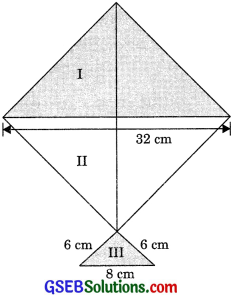Solution:
= 2 x $$\left(\frac{1}{2} \times 16 \times 16\right)$$ = 256 cm2
Similarly, area of paper of shade-II = 256 cm2
For area of paper of shade-III
a = 8cm, b = 6cm, c = 6cm
s = $$\frac{a + b + c}{2}$$ = $$\frac{8 + 6 + 6}{2}$$ = 10 cm

∴ Area of paper of shade-III
= $$\sqrt{s(s-a)(s-b)(s-c)}$$
= $$\sqrt{10(10-8)(10-6)(10-6)}$$
= $$\sqrt{10(2)(4)(4)}$$ = 8 $$\sqrt{5}$$ = 17.89 cm2.Question 8.
A floral design on a floor is made up of 16 tiles which are triangular, the sides of the triangle being 9 cm, 28 cm and 35 cm. Find the cost of polishing the tiles at the rate of 50 paise per cm2.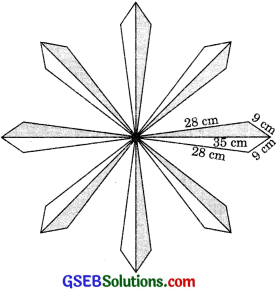Solution:
For one tile
a = 9cm, b = 28cm, c = 35cm
s = $$\frac{a + b + c}{2}$$ = $$\frac{9 + 28 + 35}{2}$$ = 36 cm

∴ Area of one tile = $$\sqrt{s(s-a)(s-b)(s-c)}$$
= $$\sqrt{36(36-9)(36-28)(36-35)}$$
= $$\sqrt{36(27)(8)(1)}$$
= $$\sqrt{36(9 \times 3)(4 \times 2)}$$
= 6 x 3 x 2$$\sqrt{6}$$ = 36$$\sqrt{6}$$cm2

∴ Area of 16 tiles
= 36$$\sqrt{6}$$ x 16 = 576$$\sqrt{6}$$ cm2
∴ Cost of polishing the tiles at the rate of 50 paise per cm2.
= 576 $$\sqrt{6}$$ x 5o p = ₹ $$\frac{576 \sqrt{6} \times 50}{100}$$
= ₹ 288$$\sqrt{6}$$ = ₹ 705.60.Question 9.
A field is in the shape of a trapezium whose parallel sides are 25 m and 10 m. The nonparallel sides are 14 m and 13 m. Find the area of the field.
Solution:
Let the given field be in the shape of a trapezium ABCD in which AB = 25 m, CD = 10 m, BC = 13m and AD = 14m. FromD,drawDEIIBC meeting AB at E. Also, draw DE .L AB.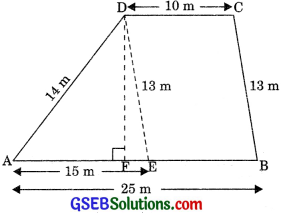∴ DE = BC = 13m
AE = AB – EB = AB – DC
= 25 – 10 = 15m
For ABD
a = 14m, b = 13m, c = 15m
S = $$\frac{a + b + c}{2}$$ = $$\frac{14 + 13 + 15}{2}$$ = $$\frac{42}{2}$$ = 21 m

∴ Area of the ΔAED
= $$\sqrt{s(s-a)(s-b)(s-c)}$$
= $$\sqrt{21(21-14)(21-13)(21-15)}$$
= $$\sqrt{21(7)(8)(6)}$$
= $$\sqrt{(7 \times 3)(7)(4 \times 2)(2 \times 3)}$$
= 7 x 3 x 2 x 2 = 84m2
∴ $$\frac{1}{2}$$ x AE x DF = 84
= $$\frac{1}{2}$$ x 15 x DF = 84
DF = $$\frac{84 x 2}{15}$$
DE = $$\frac{56}{5}$$ = 11.2m
Height of the trapezium is 11.2 m
Area of parallelogram EBCD = Base x Height
= EB x DE = 10 x $$\frac{56}{5}$$ =112m2
∴ Area of the field = Area of ΔAED + Area of parallelogram EBCD
= 84 m2 + 112 m2 = 196 m2.